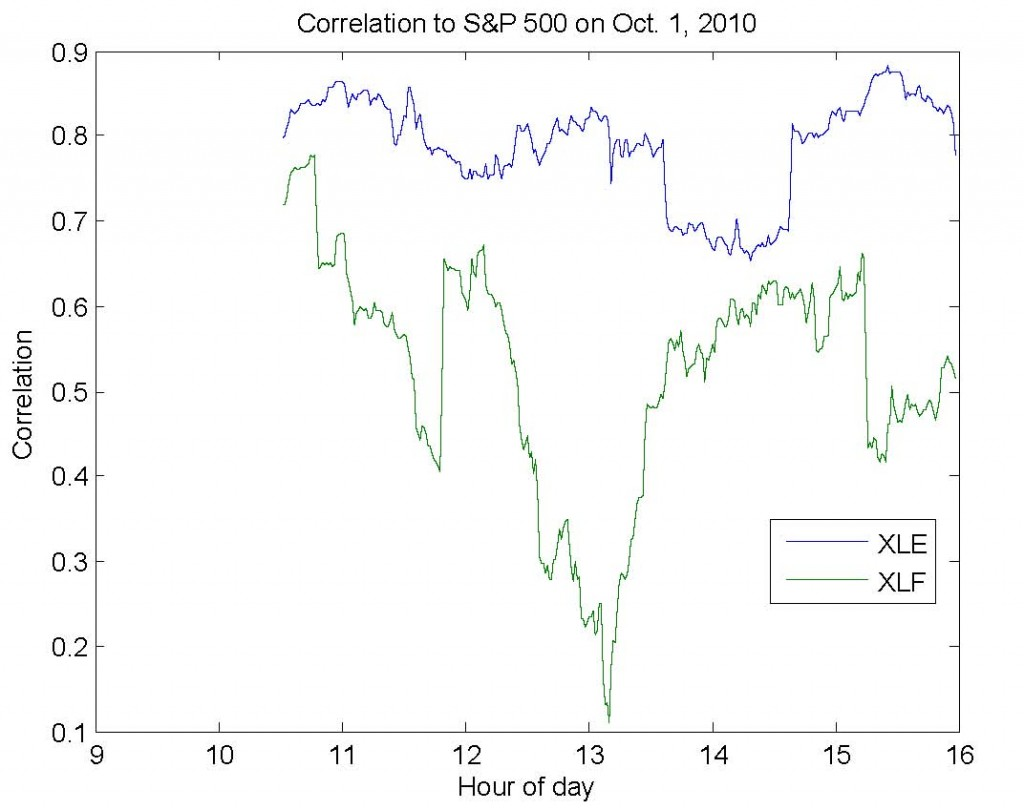# Calculating Moving Correlation in Matlab

Much of my research focuses on the dynamic relationships between assets in the market (#1,#2,#3).  Typically, I use correlation as a measure of relationship dependence since its results are easy to communicate and understand (as opposed to mutual information, which is somewhat less used in finance than it is in information theory).  However, analyzing the dynamics of correlation require us to calculate a moving correlation (a.k.a. windowed, trailing, or rolling).

Moving averages are well-understood and easily calculated – they take into account one asset at a time and produce one value for each time period.  Moving correlations, unlike moving averages,  must take into account multiple assets and produce a matrix of values for each time period.  In the simplest case, we care about the correlation between two assets – for example, the S&P 500  (SPY) and the financial sector (XLF).  In this case, we need only pay attention to one value in the matrix.  However, if we were to add the energy sector (XLE), it becomes more difficult to efficiently calculate and represent these correlations.  This is always true for 3 or more different assets.

I’ve written the code below to simplify this process (download).  First, you provide a matrix (dataMatrix) with variables in the columns – for example, SPY in column 1, XLF in column 2, and XLE in column 3.  Second, you provide a window size (windowSize).  For example, if dataMatrix contained minutely returns, then a window size of 60 would produce trailing hourly correlation estimates.  Third, you indicate which column (indexColumn) you care about seeing the results for.  In our example, we would likely specify column 1, since this would allow us to observe the correlation between (1) the S&P and financial sector and (2) the S&P and energy sector.

The image below shows the results for exactly the example above for last Friday, October 1st, 2010.### 2 Responses to “Calculating Moving Correlation in Matlab”

1. Paolo says:

Hi Michael,

it’s not clear how you deal with NA.

How would you calculate correlations for indexes across different countries where one data point can be missing due to a particular holiday in a single country?

Thanks,

Paolo

• Hi Paolo,
The code as I’ve posted doesn’t deal with NaNs gracefully. You can see from this Matlab documentation page that you can add “‘rows’, ‘complete’” to the corrcoef command to gracefully deal with the issue.
http://www.mathworks.com/help/techdoc/ref/corrcoef.html

The other alternatives are to drop that date completely, interpolate, or use a more sophisticated method for dealing with missing observations.